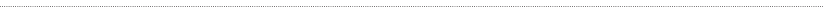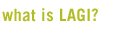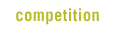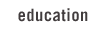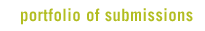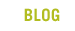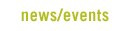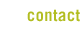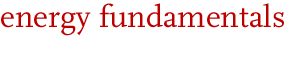Energy is in everything.

The word “electricity” comes from the Greek “ήλεκτρον” which literally means “amber-like.” It was observed in ancient times that the rubbing of amber would cause light objects like feathers to adhere to the surface. We now know that this is cause by a buildup of electrical energy on the surface of an insulator (static electricity).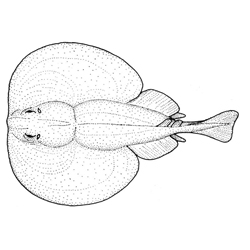Evidence that 15th C. Arab scientists understood electricity at a sophisticated level is that the word for the electric ray fish coined at the time is the same as the word for lightning (raad/رعد).

Energy cannot be created or gotten rid of, but it can change its form.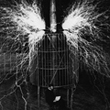Its form can be either potential (static at observable scale/position) or kinetic (dynamic/movement).

Its form can also be either thermal, sonic, gravitational, chemical, nuclear, mechanical, elastic, magnetic, radiant, or electrical.

It can be measured in JOULES, CALORIES, BTUs, or KILLOWAT-HOURS (these are all measures of the same thing much like inches and centimeters are both measures of distance). You may also see energy measured in tonnes of oil equivalent (TOE), barrels of oil equivalent (BOE), or cubic feet of natural gas equivalent, but these are approximations since the energy content of oil and gas can vary per volume based on many variables. You can see how to shift between these measures on the CONVERSIONS page.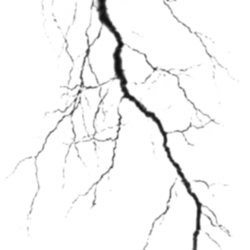We can trace electrical energy (E) to the subatomic level where particles hold charges. We call them either positive or negative (a convention that has its origins in the work of Benjamin Franklin). We measure the strength of a charge in Columbs (C). A single electron has a “negative” charge of 1.6022x10-19 Columbs.

When there is a charge in one object in relation to another object, this creates a potential difference in charge that we measure in Volts (V). It is this Voltage that causes the electrons to move from one object to the other in order to equalize the charges (correct the local imbalance). Electrical energy is equal to Columbs x Voltage (E = CV). A greater charge and/or a greater difference in charge between two objects creates an increase in electrical energy. At this point it is still potential energy.The actual flow (the kinetic form) of the electrons is known as Current and is measured in Amperes (I). Because it is a directional flow (one object to another differently charged object),it is measured over time (t). The amount of flow per quantity of time is Power (P), measured in the unit called watts.
This is important to understand because there is often confusion between measures of Power (watts) and measures of Energy (watt-hours).

Power (P) is a measure that can tell us the capacity of a system. It is equal to Voltage x Amperage (P = V I). It tells us both how much potential charge differential (V) and how strong a current (I) a particular system can accommodate. For example, an electrical system such as a toaster can safely function below its power rating or at that power rating, but if the power exceeds, it will lead to energy transfer in the form of heat. Raising either the Voltage or the Amperage will increase the Power.So if you have a 30 Ampere toaster that runs on a 110 Volt circuit (North American), it will draw 3,300 watts. If you plug that same toaster into a 240 Volt circuit (European), it will draw 6,600 watts and the circuit breaker will snap to disconnect the flow of energy, otherwise heat buildup could cause a fire.

The last thing to understand about basic electrical power is that conducting materials (that stuff which the electrons flow through, either copper wire or your body) are not perfect conductors. Along the way, some of the electrical energy is always lost to heat. This is Resistance (R) and it is measured in Ohms.Thinking about water flow through a hose is a good way to understand resistance. Assuming a constant water pressure (Voltage equivalent with electricity in the analogy), water will flow slower through a long hose than through a short hose. This is due to the friction in the hose which offers resistance to the current. If you know Voltage (V) and Resistance (R), you can determine Current (I): I = V/R. A resistor in a circuit has a rating in Ohms (Ω) and is diagrammed with a zig-zag line.

Here are some useful relationships between the variables that we’ve discussed so far:

E = VC = VIt = Pt = V2 t/R = I2 Rt
Electrical Energy = Voltage x Charge = Voltage x Current x Time = Power x Time = (Voltage squared x Time)/Resistance = Current squared x Resistance x Time

P = VI
Watts = Volts x Amperes